Скачать презентацию B 280 F Introduction to Financial Management Lecture

fafb137e3a2a62c3cd4057029e8fa956.ppt

• Количество слайдов: 65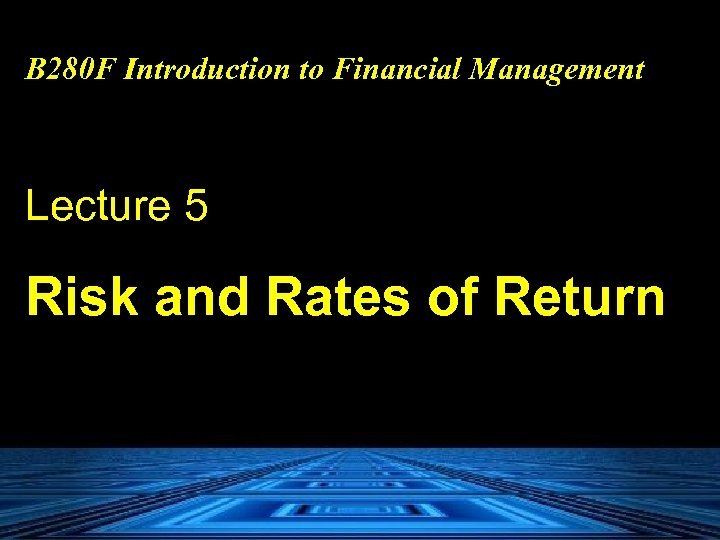B 280 F Introduction to Financial Management Lecture 5 Risk and Rates of Return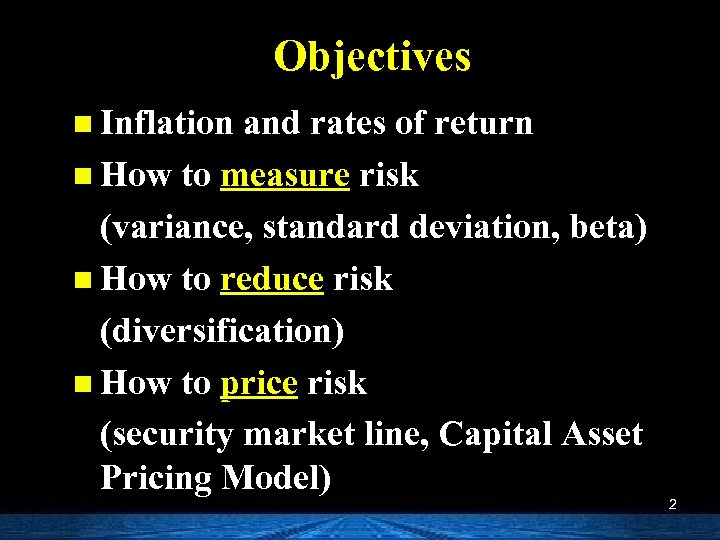Objectives n Inflation and rates of return n How to measure risk (variance, standard deviation, beta) n How to reduce risk (diversification) n How to price risk (security market line, Capital Asset Pricing Model) 2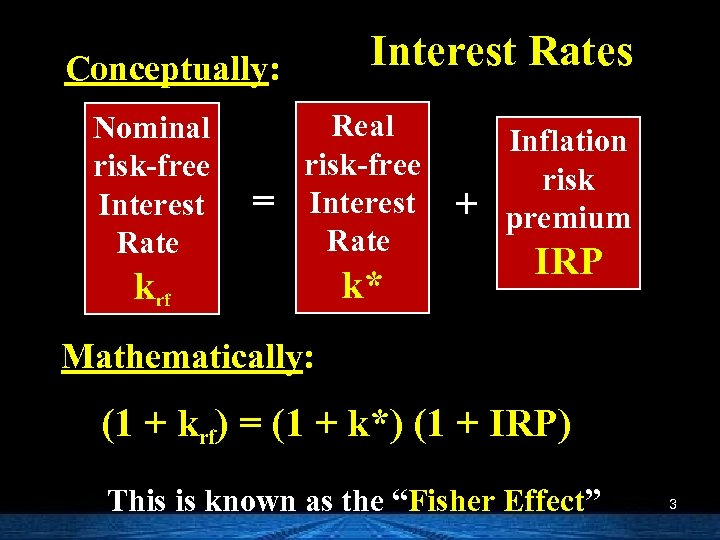Interest Rates Conceptually: Nominal risk-free Interest Rate = Real risk-free Interest Rate krf k* + Inflation risk premium IRP Mathematically: (1 + krf) = (1 + k*) (1 + IRP) This is known as the “Fisher Effect” 3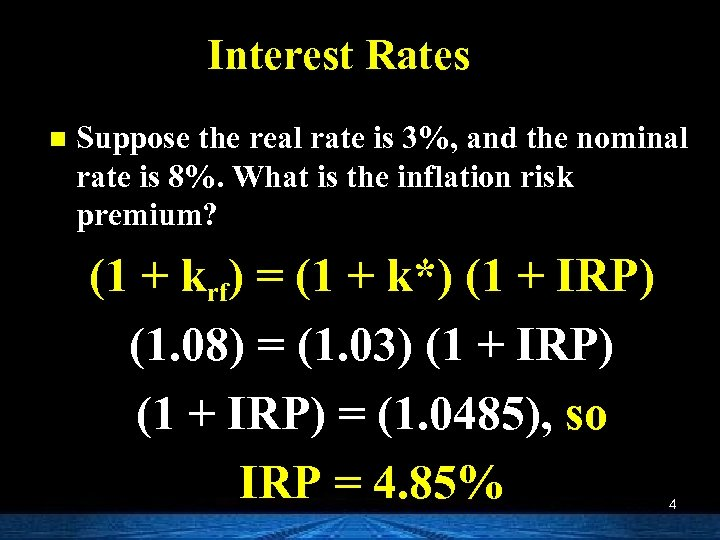Interest Rates n Suppose the real rate is 3%, and the nominal rate is 8%. What is the inflation risk premium? (1 + krf) = (1 + k*) (1 + IRP) (1. 08) = (1. 03) (1 + IRP) = (1. 0485), so IRP = 4. 85% 4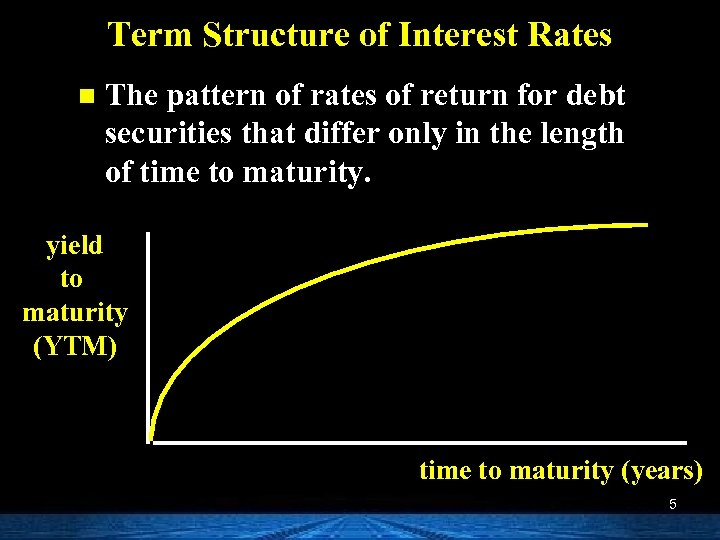Term Structure of Interest Rates n The pattern of rates of return for debt securities that differ only in the length of time to maturity. yield to maturity (YTM) time to maturity (years) 5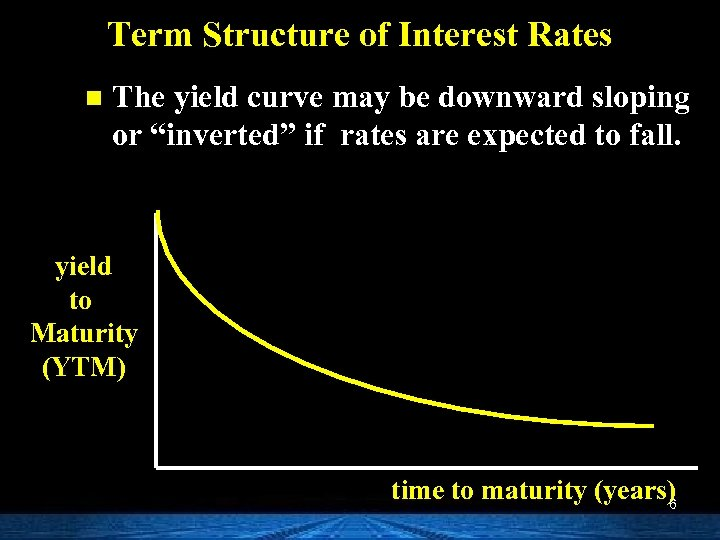Term Structure of Interest Rates n The yield curve may be downward sloping or “inverted” if rates are expected to fall. yield to Maturity (YTM) time to maturity (years) 6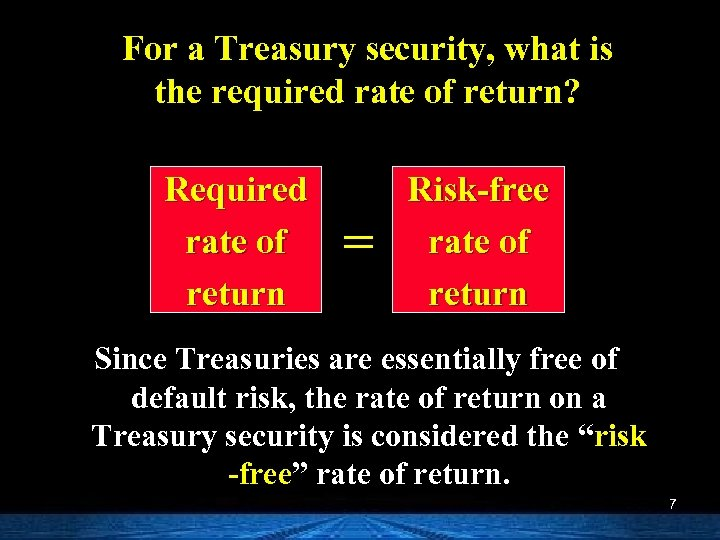For a Treasury security, what is the required rate of return? Required rate of return = Risk-free rate of return Since Treasuries are essentially free of default risk, the rate of return on a Treasury security is considered the “risk -free” rate of return. 7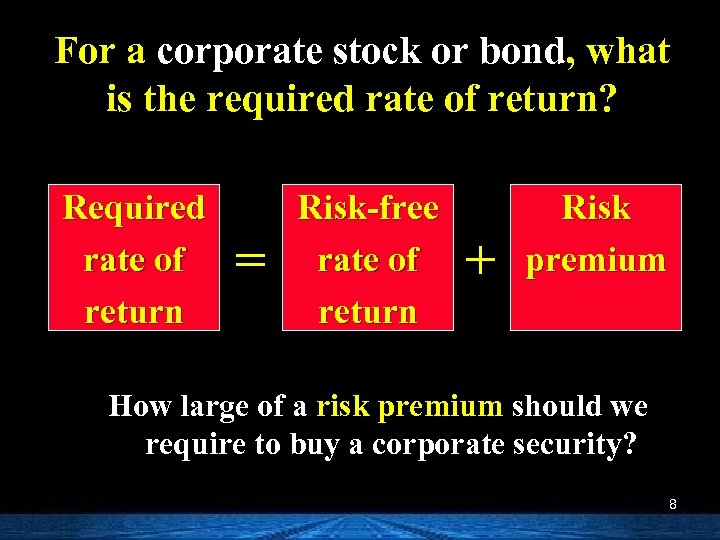For a corporate stock or bond, what is the required rate of return? Required rate of return = Risk-free rate of return + Risk premium How large of a risk premium should we require to buy a corporate security? 8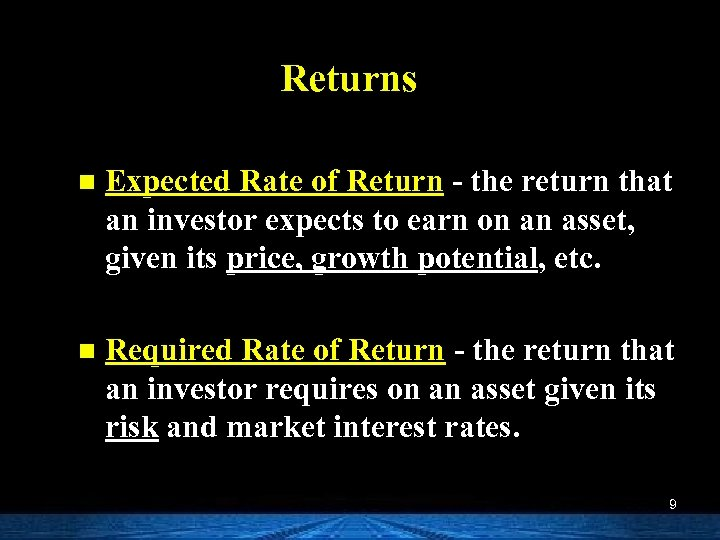Returns n Expected Rate of Return - the return that an investor expects to earn on an asset, given its price, growth potential, etc. n Required Rate of Return - the return that an investor requires on an asset given its risk and market interest rates. 9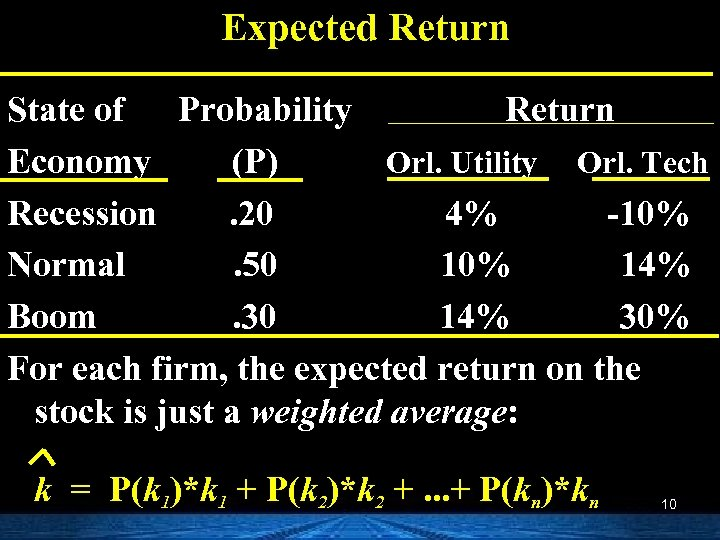Expected Return State of Probability Return Economy (P) Orl. Utility Orl. Tech Recession. 20 4% -10% Normal. 50 10% 14% Boom. 30 14% 30% For each firm, the expected return on the stock is just a weighted average: k = P(k 1)*k 1 + P(k 2)*k 2 +. . . + P(kn)*kn 10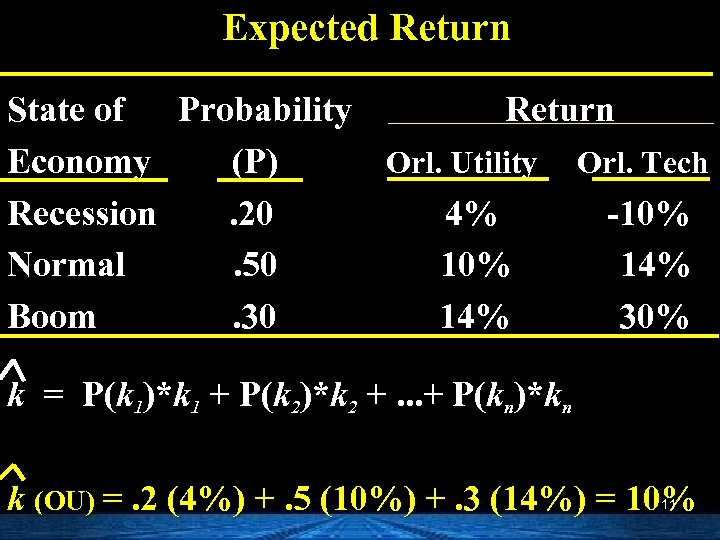Expected Return State of Probability Return Economy (P) Orl. Utility Orl. Tech Recession. 20 4% -10% Normal. 50 10% 14% Boom. 30 14% 30% k = P(k 1)*k 1 + P(k 2)*k 2 +. . . + P(kn)*kn k (OU) =. 2 (4%) +. 5 (10%) +. 3 (14%) = 10% 11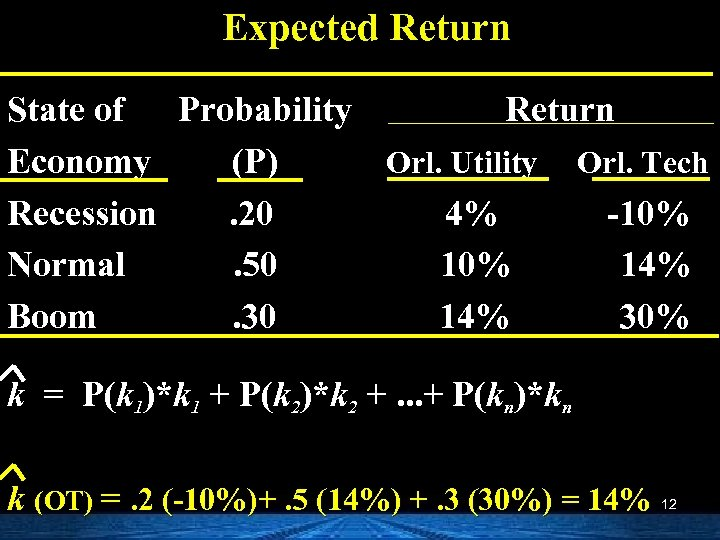Expected Return State of Probability Return Economy (P) Orl. Utility Orl. Tech Recession. 20 4% -10% Normal. 50 10% 14% Boom. 30 14% 30% k = P(k 1)*k 1 + P(k 2)*k 2 +. . . + P(kn)*kn k (OT) =. 2 (-10%)+. 5 (14%) +. 3 (30%) = 14% 12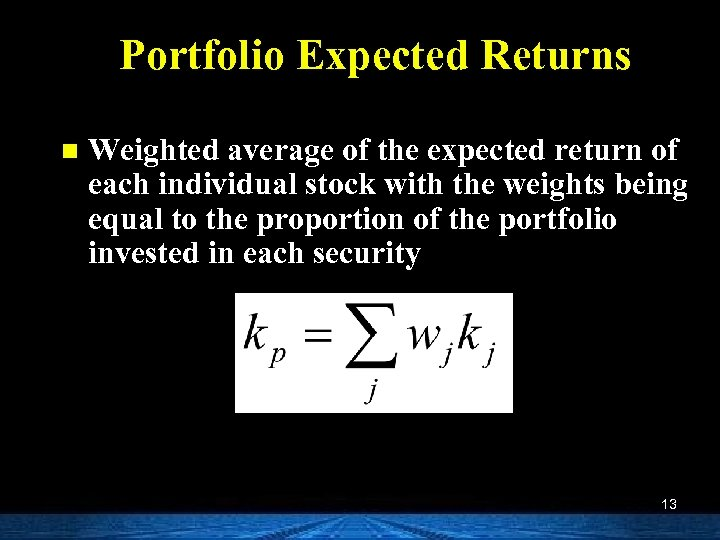Portfolio Expected Returns n Weighted average of the expected return of each individual stock with the weights being equal to the proportion of the portfolio invested in each security 13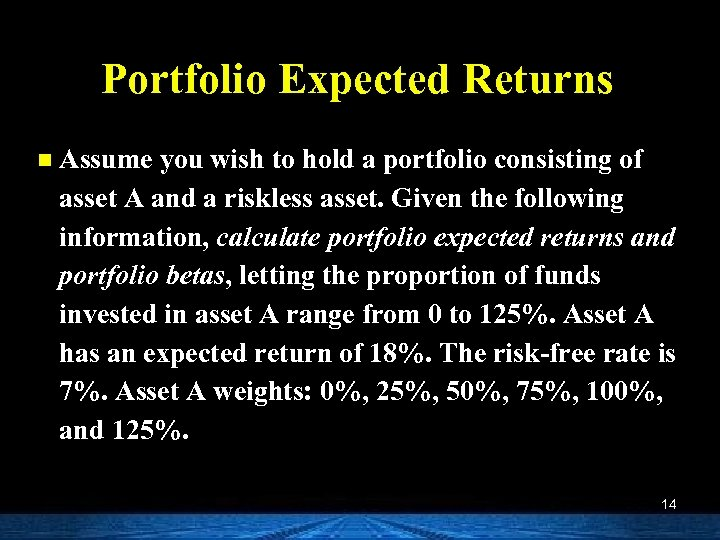Portfolio Expected Returns n Assume you wish to hold a portfolio consisting of asset A and a riskless asset. Given the following information, calculate portfolio expected returns and portfolio betas, letting the proportion of funds invested in asset A range from 0 to 125%. Asset A has an expected return of 18%. The risk-free rate is 7%. Asset A weights: 0%, 25%, 50%, 75%, 100%, and 125%. 14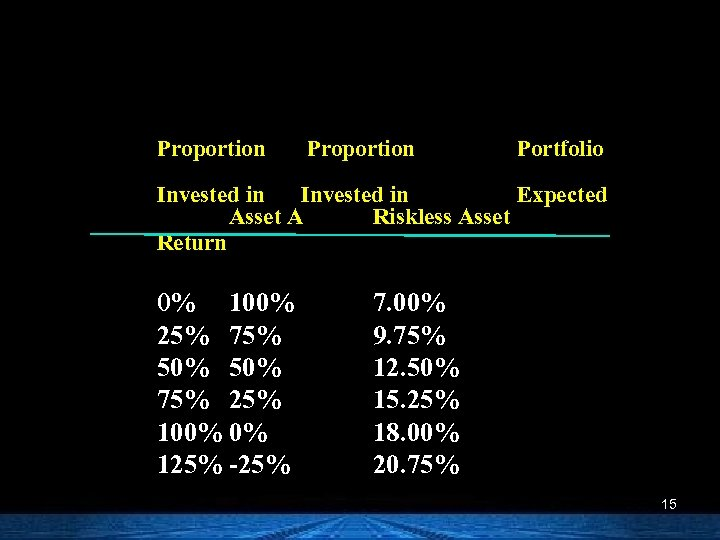Proportion Portfolio Invested in Expected Asset A Riskless Asset Return 0% 100% 25% 75% 50% 75% 25% 100% 0% 125% -25% 7. 00% 9. 75% 12. 50% 15. 25% 18. 00% 20. 75% 15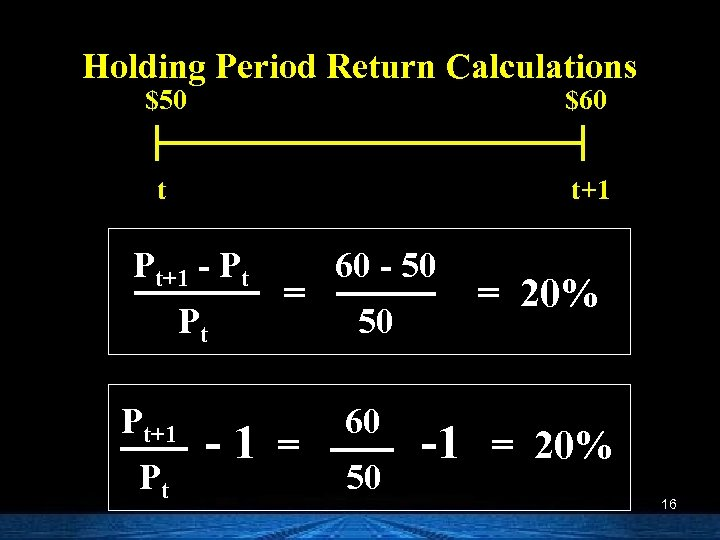Holding Period Return Calculations \$50 \$60 t t+1 Pt+1 - Pt Pt Pt+1 Pt = -1 = 60 - 50 50 60 50 = 20% -1 = 20% 16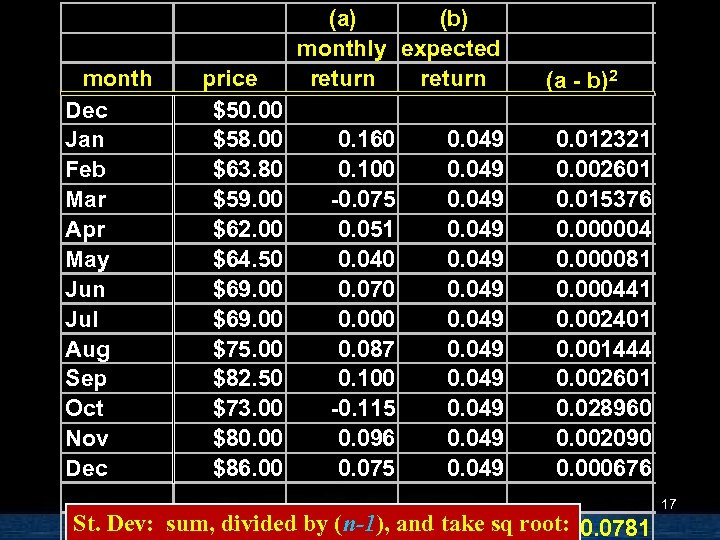month Dec Jan Feb Mar Apr May Jun Jul Aug Sep Oct Nov Dec price \$50. 00 \$58. 00 \$63. 80 \$59. 00 \$62. 00 \$64. 50 \$69. 00 \$75. 00 \$82. 50 \$73. 00 \$80. 00 \$86. 00 (a) (b) monthly expected return 0. 160 0. 100 -0. 075 0. 051 0. 040 0. 070 0. 000 0. 087 0. 100 -0. 115 0. 096 0. 075 0. 049 0. 049 (a - b)2 0. 012321 0. 002601 0. 015376 0. 000004 0. 000081 0. 000441 0. 002401 0. 001444 0. 002601 0. 028960 0. 002090 0. 000676 St. Dev: sum, divided by (n-1), and take sq root: 0. 0781 17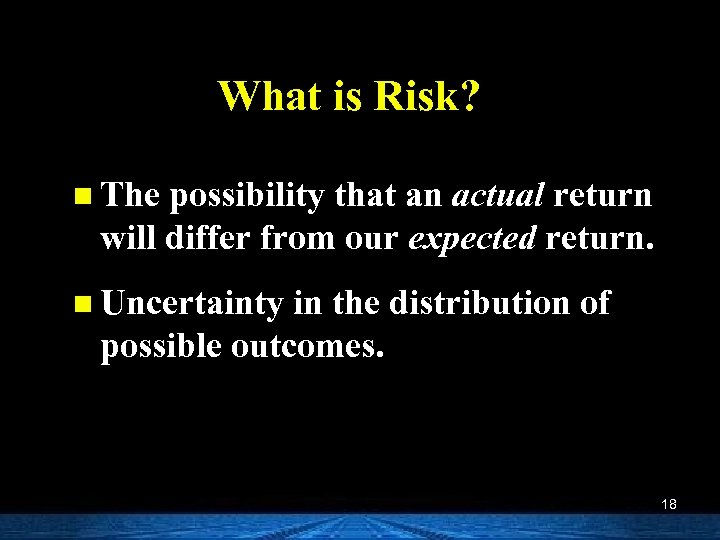What is Risk? n The possibility that an actual return will differ from our expected return. n Uncertainty in the distribution of possible outcomes. 18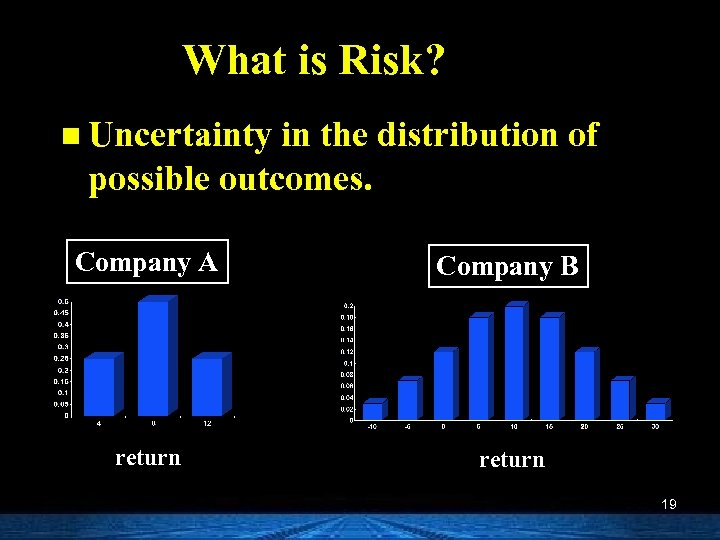What is Risk? n Uncertainty in the distribution of possible outcomes. Company A Company B return 19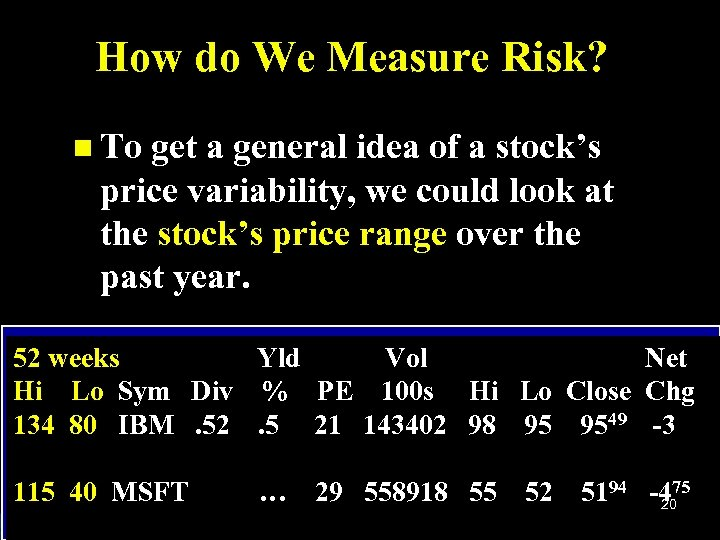How do We Measure Risk? n To get a general idea of a stock’s price variability, we could look at the stock’s price range over the past year. 52 weeks Yld Vol Net Hi Lo Sym Div % PE 100 s Hi Lo Close Chg 134 80 IBM. 52. 5 21 143402 98 95 9549 -3 115 40 MSFT … 29 558918 55 52 5194 -475 20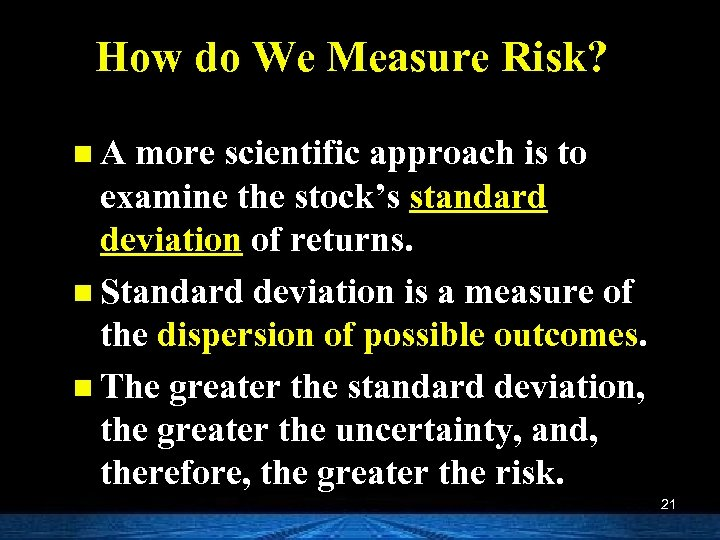How do We Measure Risk? n A more scientific approach is to examine the stock’s standard deviation of returns. n Standard deviation is a measure of the dispersion of possible outcomes. n The greater the standard deviation, the greater the uncertainty, and, therefore, the greater the risk. 21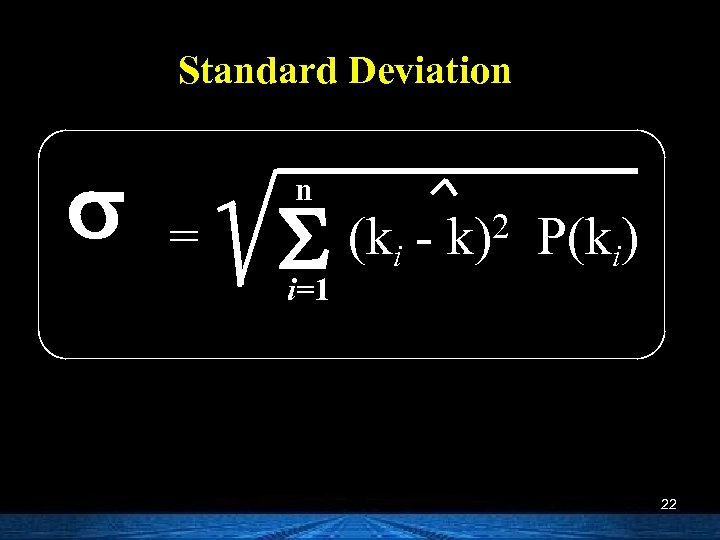Standard Deviation s = n S i=1 (ki - 2 k) P(ki) 22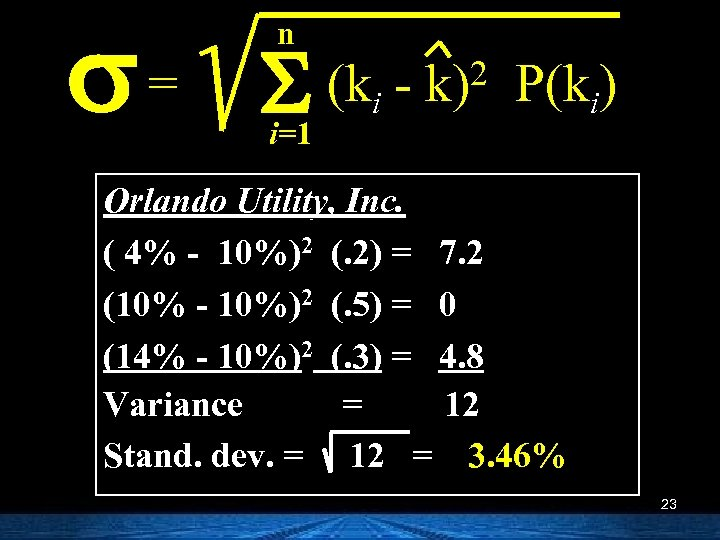s= n S (k i=1 i 2 k) P(ki) Orlando Utility, Inc. ( 4% - 10%)2 (. 2) = 7. 2 (10% - 10%)2 (. 5) = 0 (14% - 10%)2 (. 3) = 4. 8 Variance = 12 Stand. dev. = 12 = 3. 46% 23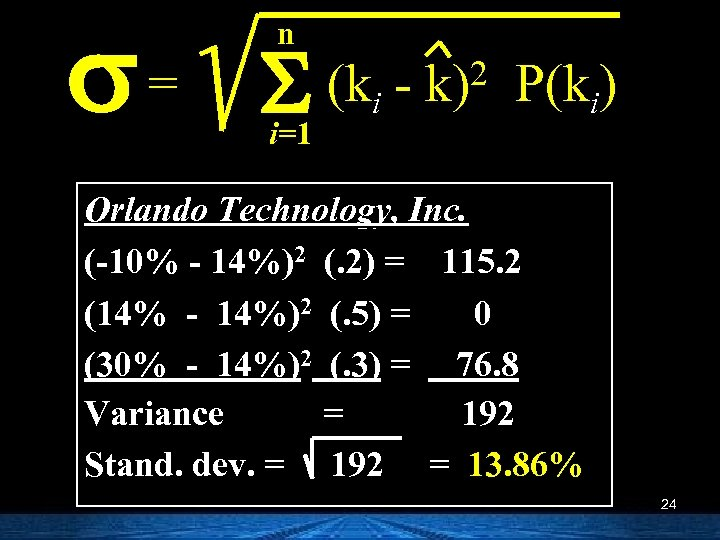s= n S (k i=1 i 2 k) P(ki) Orlando Technology, Inc. (-10% - 14%)2 (. 2) = 115. 2 (14% - 14%)2 (. 5) = 0 (30% - 14%)2 (. 3) = 76. 8 Variance = 192 Stand. dev. = 192 = 13. 86% 24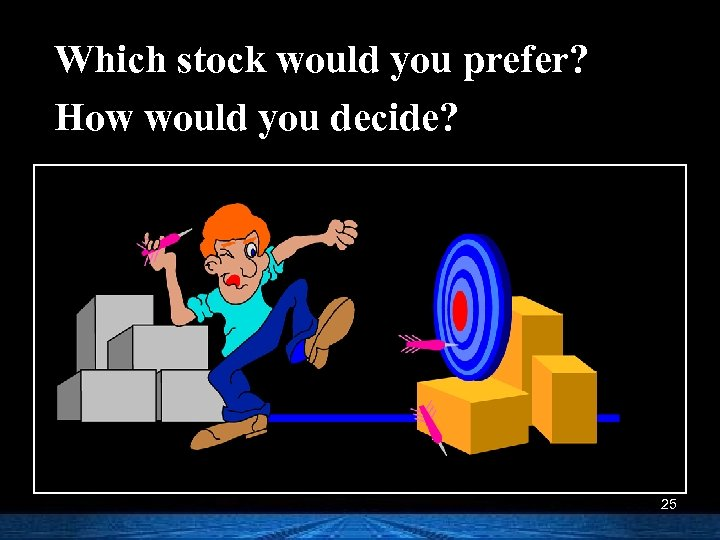Which stock would you prefer? How would you decide? 25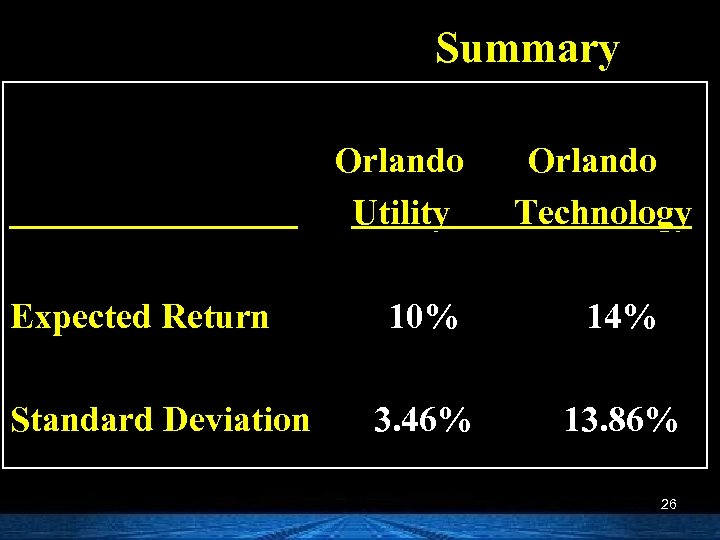Summary Orlando Utility Expected Return Standard Deviation Orlando Technology 10% 14% 3. 46% 13. 86% 26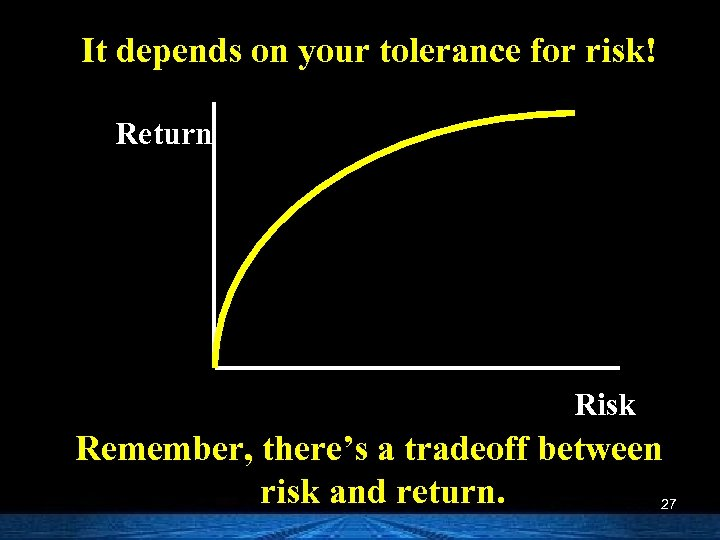It depends on your tolerance for risk! Return Risk Remember, there’s a tradeoff between risk and return. 27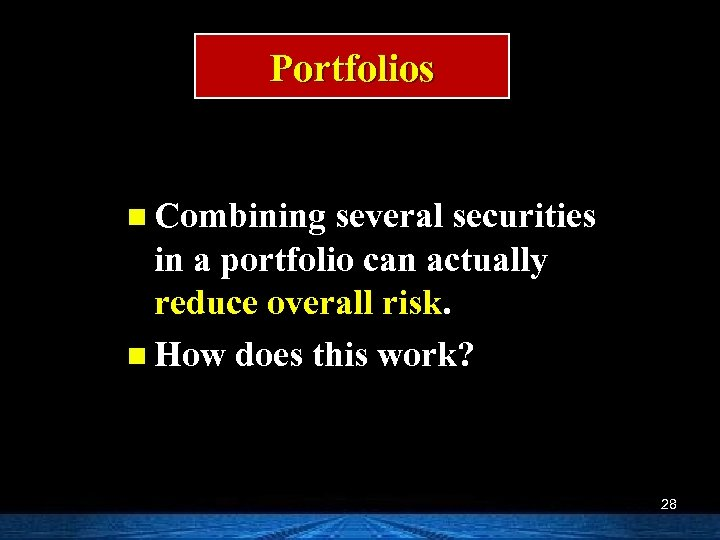Portfolios n Combining several securities in a portfolio can actually reduce overall risk. n How does this work? 28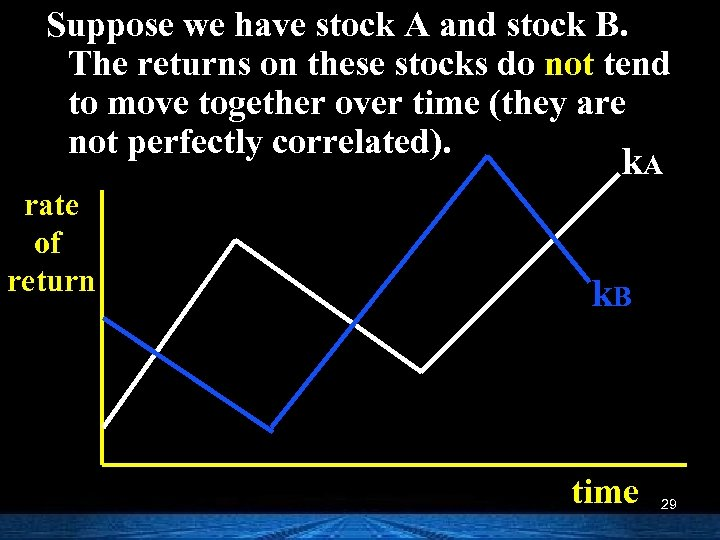Suppose we have stock A and stock B. The returns on these stocks do not tend to move together over time (they are not perfectly correlated). k. A rate of return k. B time 29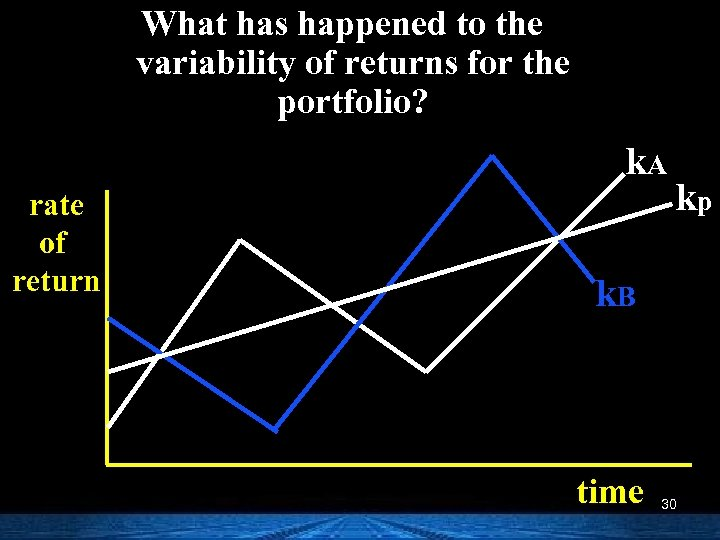What has happened to the variability of returns for the portfolio? k. A rate of return kp k. B time 30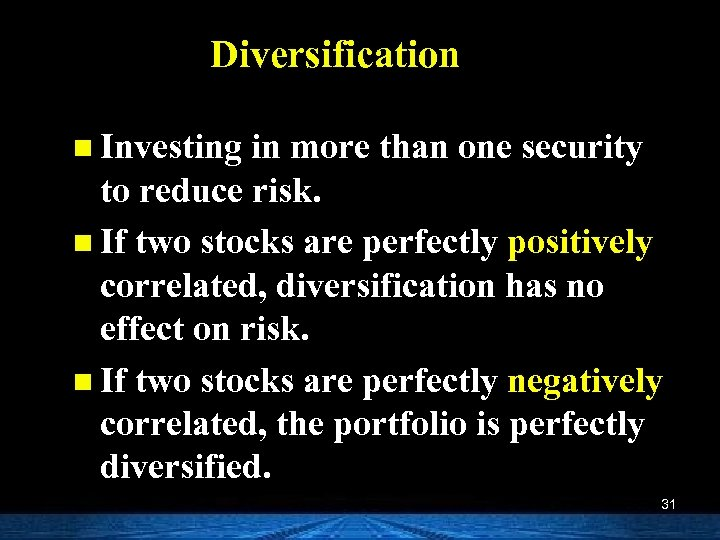Diversification n Investing in more than one security to reduce risk. n If two stocks are perfectly positively correlated, diversification has no effect on risk. n If two stocks are perfectly negatively correlated, the portfolio is perfectly diversified. 31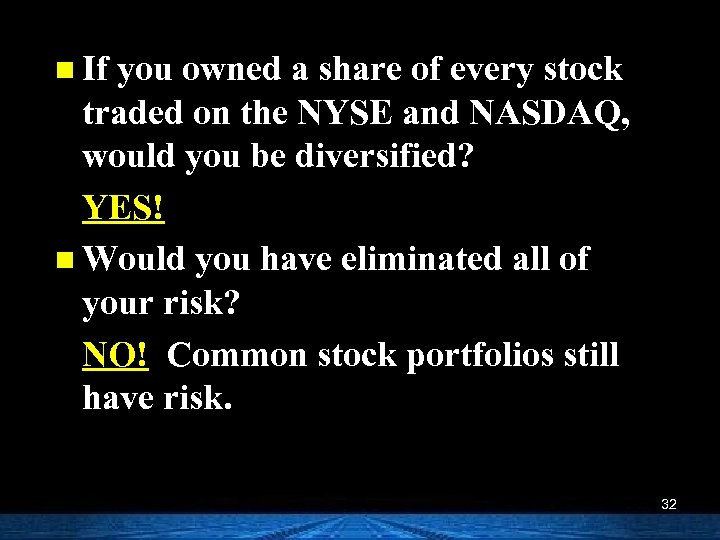n If you owned a share of every stock traded on the NYSE and NASDAQ, would you be diversified? YES! n Would you have eliminated all of your risk? NO! Common stock portfolios still have risk. 32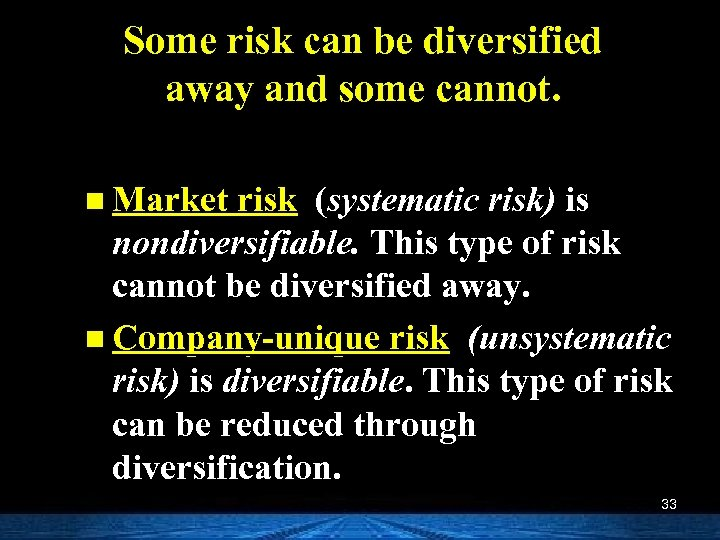Some risk can be diversified away and some cannot. n Market risk (systematic risk) is nondiversifiable. This type of risk cannot be diversified away. n Company-unique risk (unsystematic risk) is diversifiable. This type of risk can be reduced through diversification. 33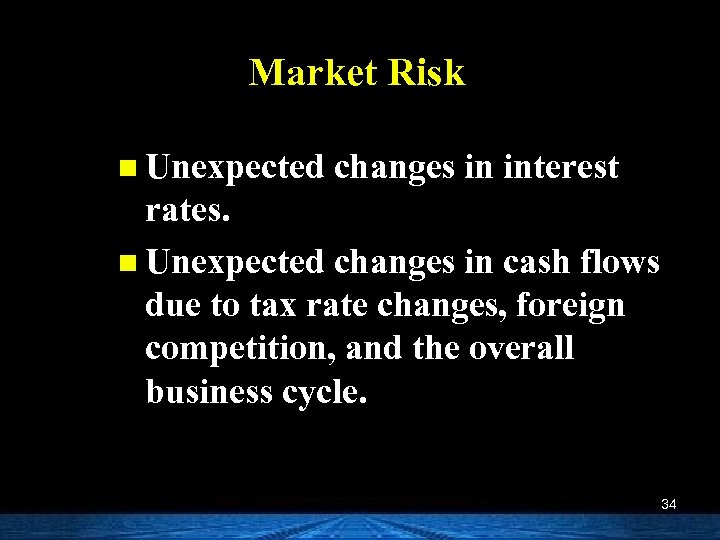Market Risk n Unexpected changes in interest rates. n Unexpected changes in cash flows due to tax rate changes, foreign competition, and the overall business cycle. 34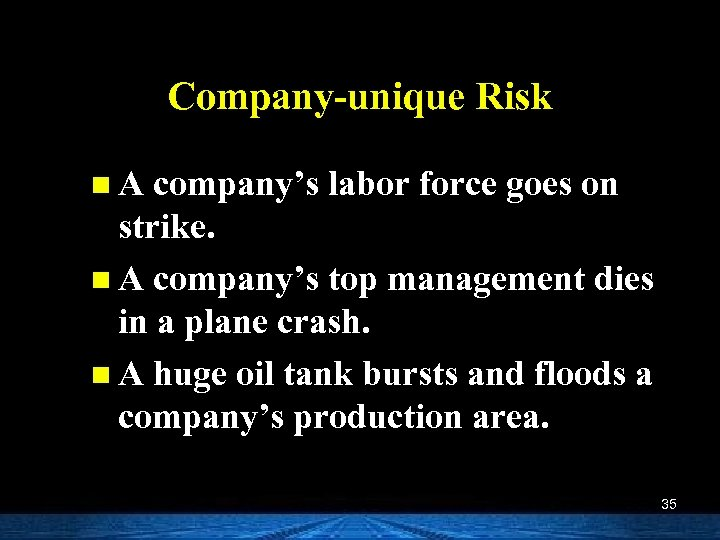Company-unique Risk n A company’s labor force goes on strike. n A company’s top management dies in a plane crash. n A huge oil tank bursts and floods a company’s production area. 35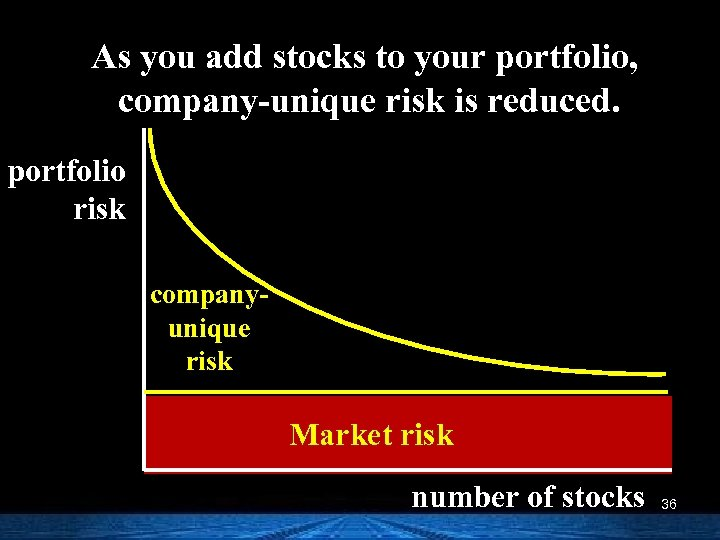As you add stocks to your portfolio, company-unique risk is reduced. portfolio risk companyunique risk Market risk number of stocks 36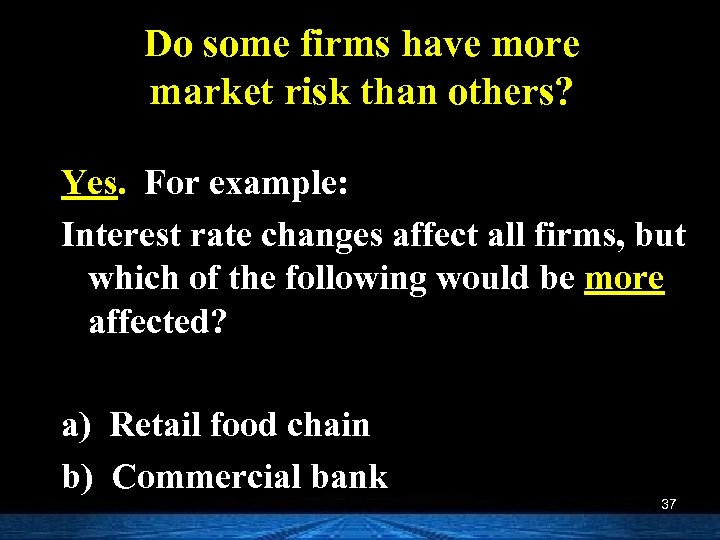Do some firms have more market risk than others? Yes. For example: Interest rate changes affect all firms, but which of the following would be more affected? a) Retail food chain b) Commercial bank 37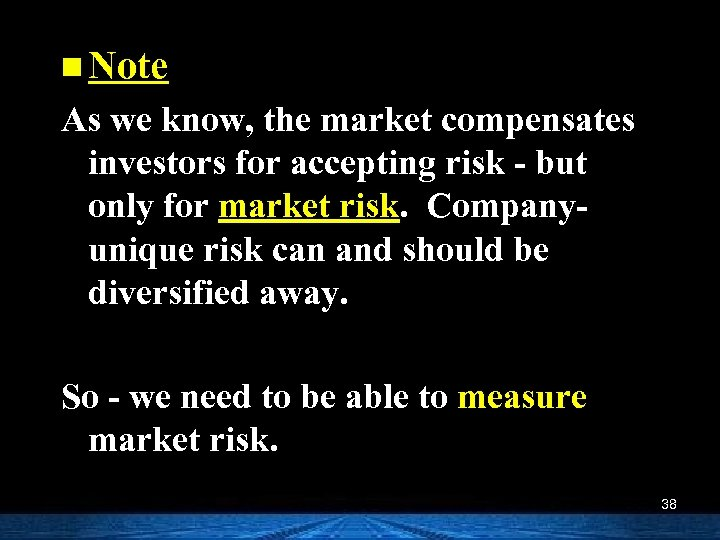n Note As we know, the market compensates investors for accepting risk - but only for market risk. Companyunique risk can and should be diversified away. So - we need to be able to measure market risk. 38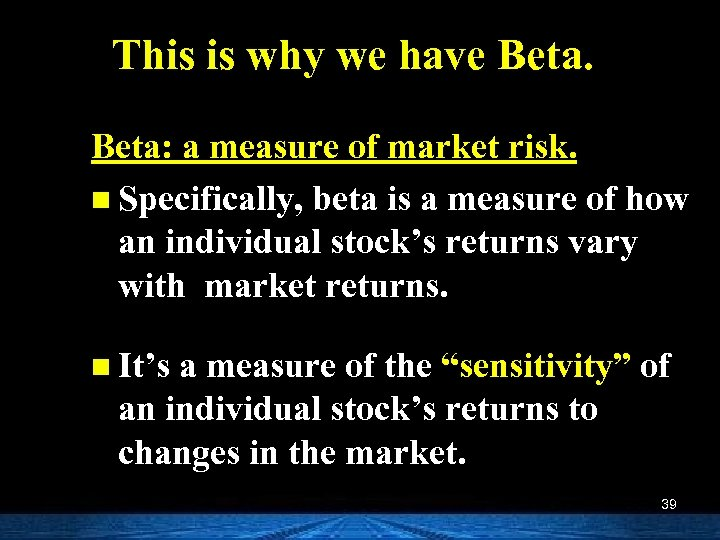This is why we have Beta: a measure of market risk. n Specifically, beta is a measure of how an individual stock’s returns vary with market returns. n It’s a measure of the “sensitivity” of an individual stock’s returns to changes in the market. 39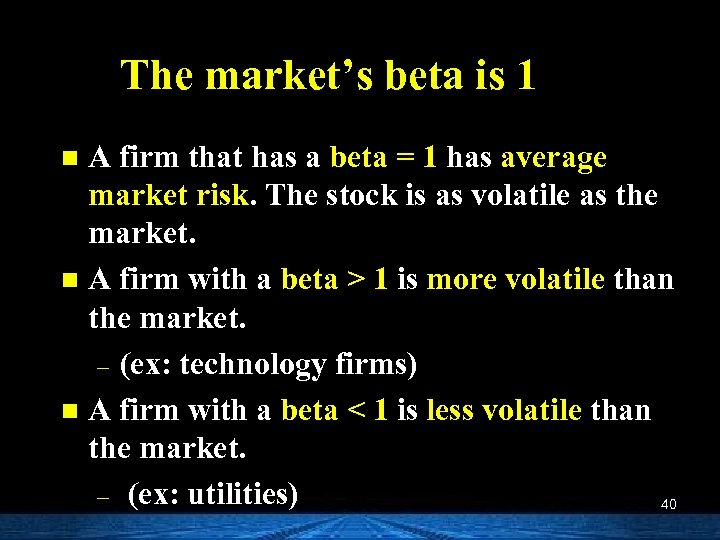The market’s beta is 1 A firm that has a beta = 1 has average market risk. The stock is as volatile as the market. n A firm with a beta > 1 is more volatile than the market. – (ex: technology firms) n A firm with a beta < 1 is less volatile than the market. – (ex: utilities) 40 n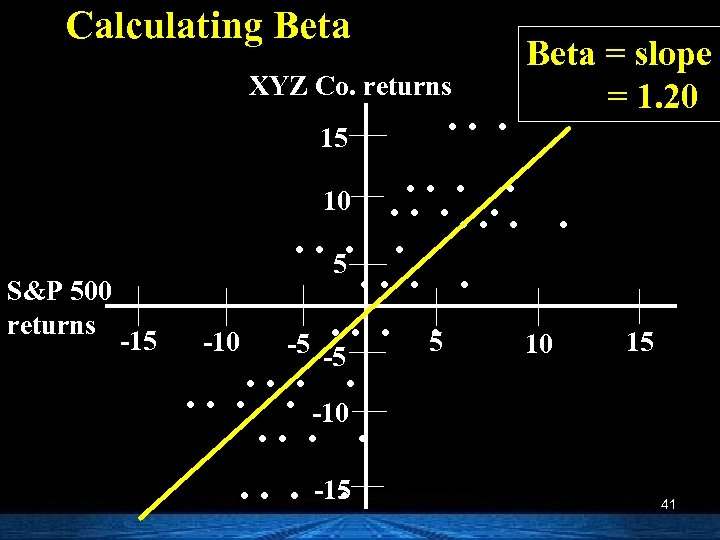Calculating Beta XYZ Co. returns 15 S&P 500 returns -15 . . . Beta = slope = 1. 20 . . 10. . . 5. -10 -5 -5 10. . . . -10. . . . -15. 15 41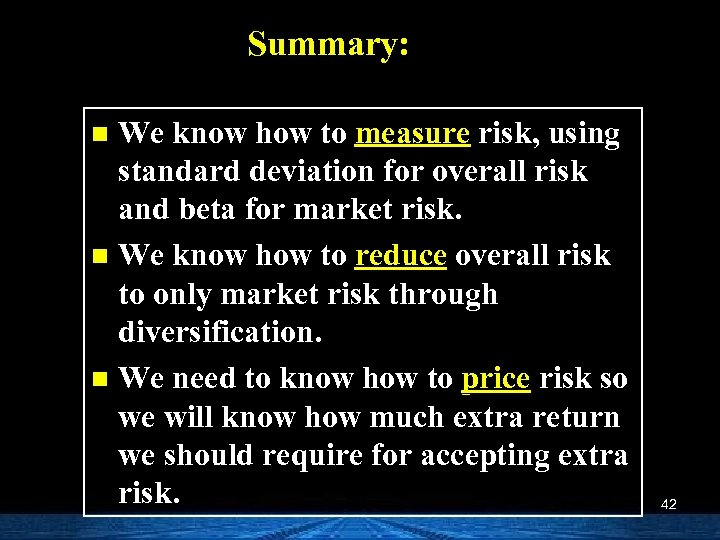Summary: We know how to measure risk, using standard deviation for overall risk and beta for market risk. n We know how to reduce overall risk to only market risk through diversification. n We need to know how to price risk so we will know how much extra return we should require for accepting extra risk. n 42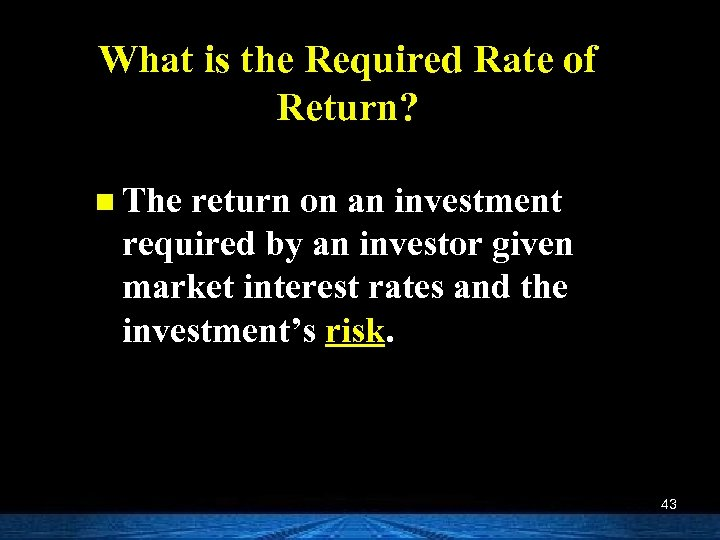What is the Required Rate of Return? n The return on an investment required by an investor given market interest rates and the investment’s risk. 43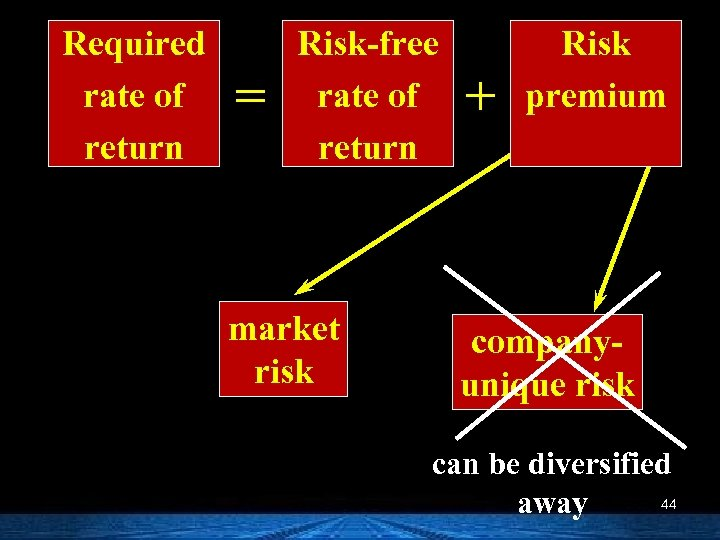Required rate of return = Risk-free rate of return market risk + Risk premium companyunique risk can be diversified 44 away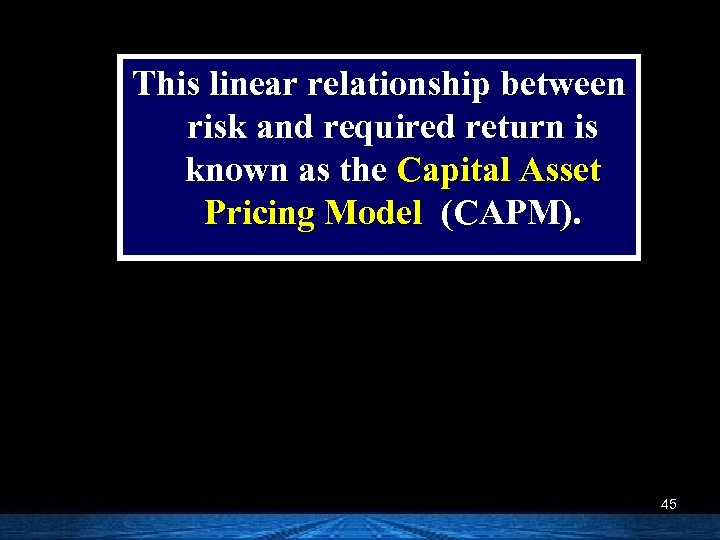This linear relationship between risk and required return is known as the Capital Asset Pricing Model (CAPM). 45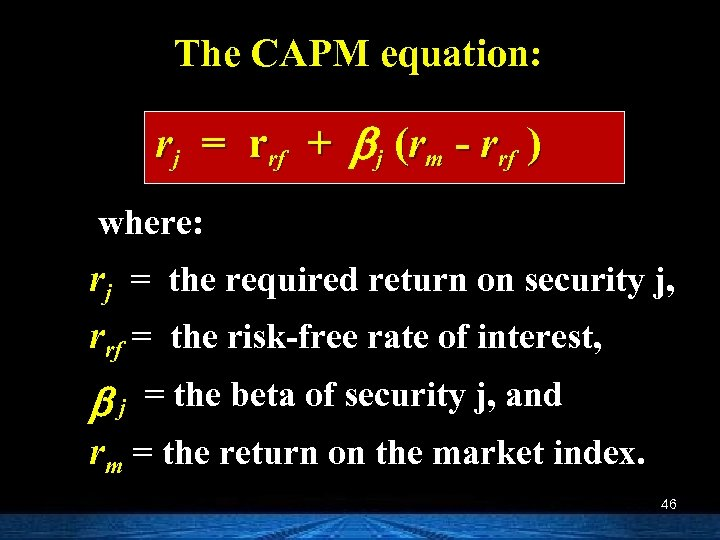The CAPM equation: rj = rrf + b j (rm - rrf ) where: rj = the required return on security j, rrf = the risk-free rate of interest, b j = the beta of security j, and rm = the return on the market index. 46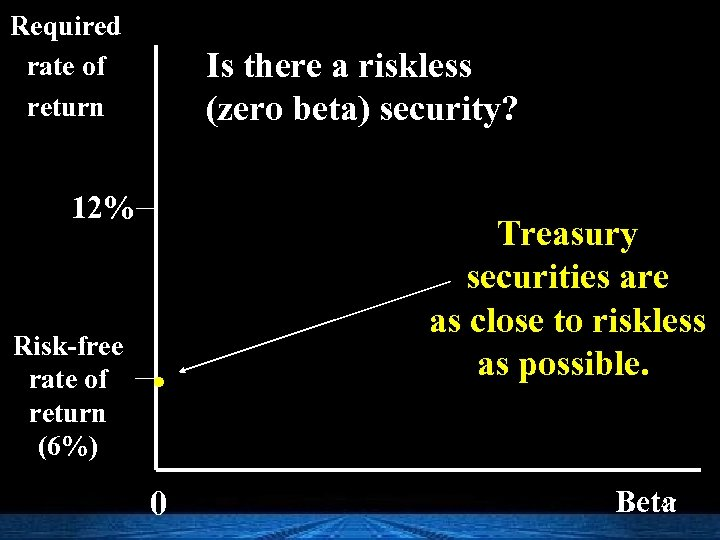Required rate of return Is there a riskless (zero beta) security? 12% Risk-free rate of return (6%) . 0 Treasury securities are as close to riskless as possible. 47 Beta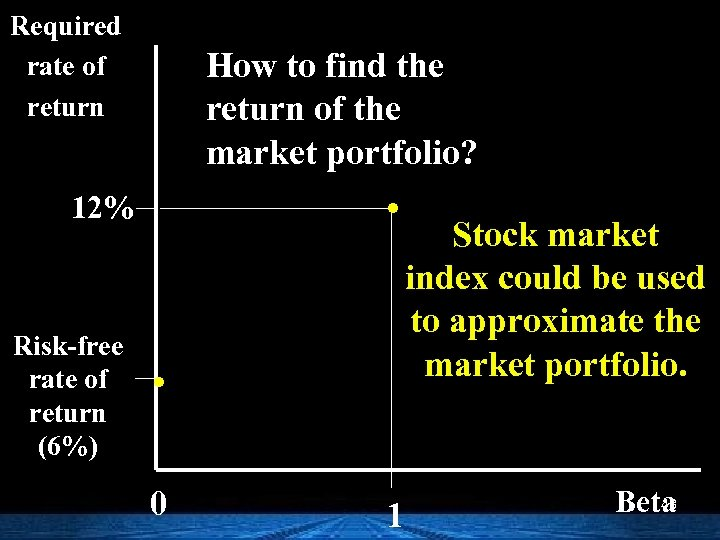Required rate of return How to find the return of the market portfolio? . 12% Risk-free rate of return (6%) . 0 1 Stock market index could be used to approximate the market portfolio. 48 Beta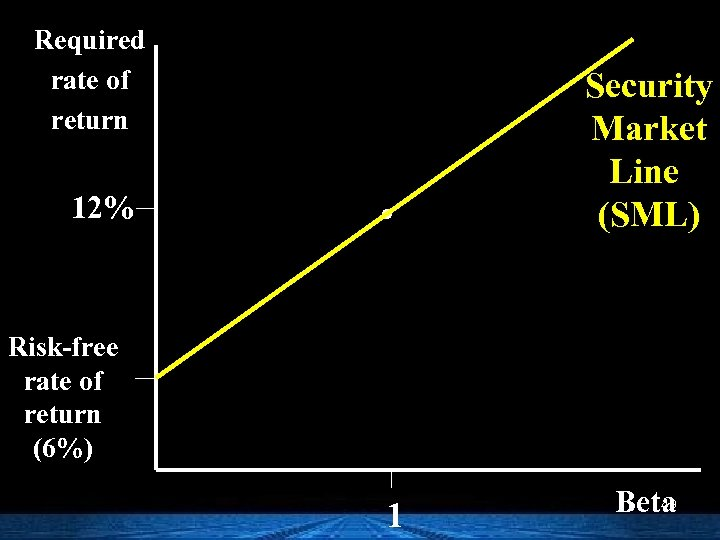Required rate of return 12% . Security Market Line (SML) Risk-free rate of return (6%) 1 49 Beta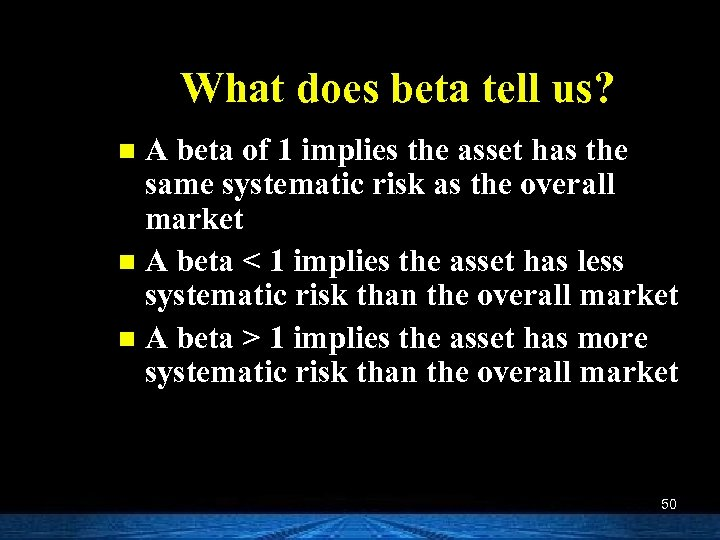What does beta tell us? A beta of 1 implies the asset has the same systematic risk as the overall market n A beta < 1 implies the asset has less systematic risk than the overall market n A beta > 1 implies the asset has more systematic risk than the overall market n 50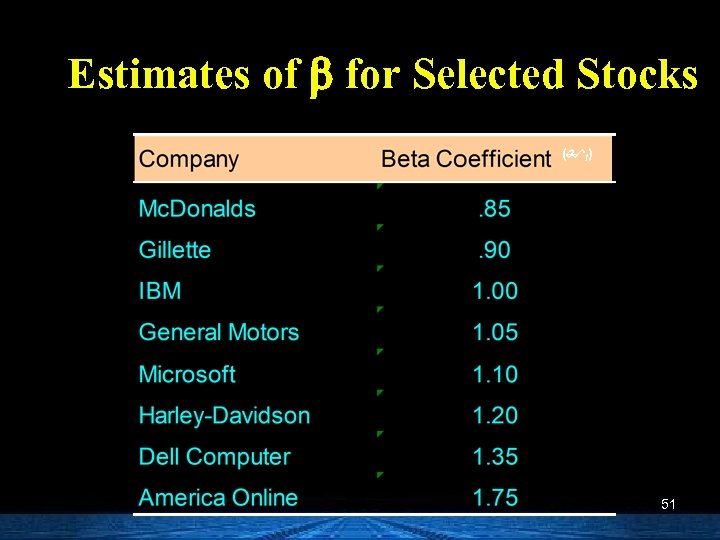Estimates of b for Selected Stocks ( I) 51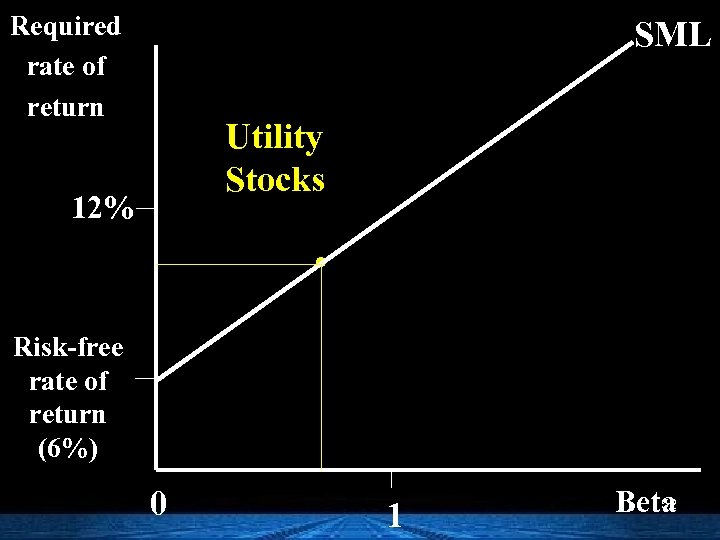Required rate of return SML Utility Stocks 12% . Risk-free rate of return (6%) 0 1 52 Beta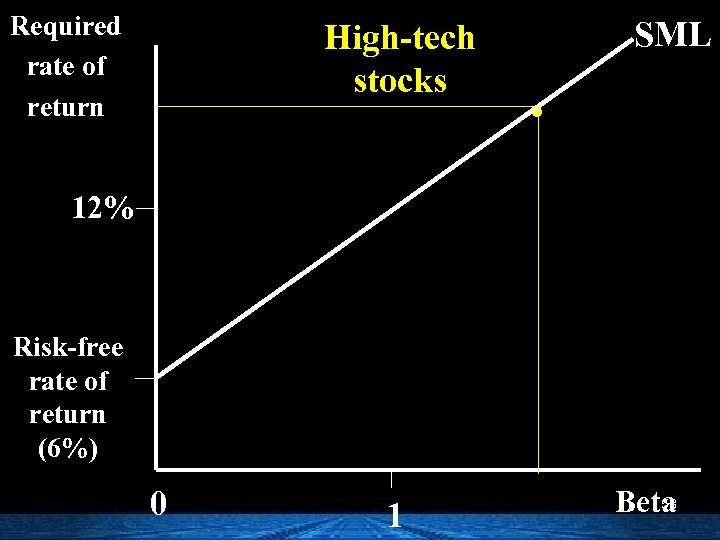Required rate of return High-tech stocks SML . 12% Risk-free rate of return (6%) 0 1 53 Beta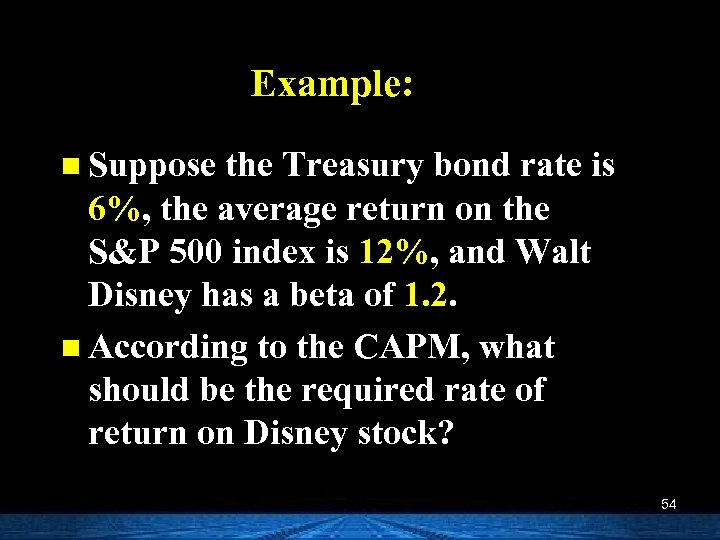Example: n Suppose the Treasury bond rate is 6%, the average return on the S&P 500 index is 12%, and Walt Disney has a beta of 1. 2. n According to the CAPM, what should be the required rate of return on Disney stock? 54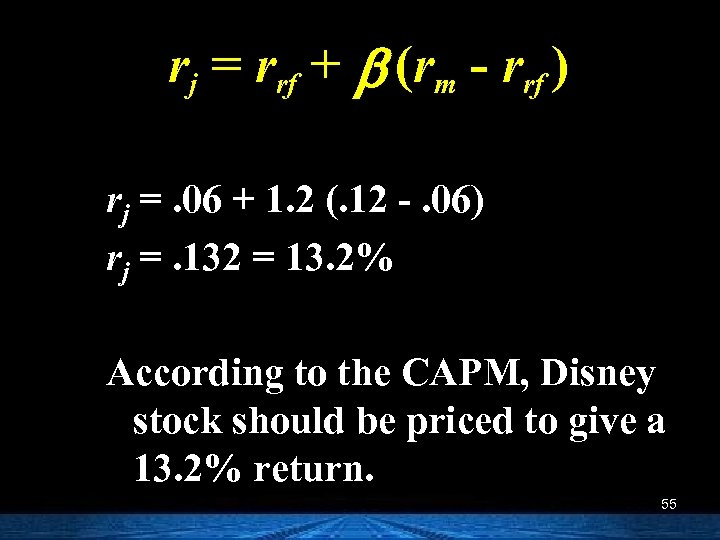rj = rrf + b (rm - rrf ) rj =. 06 + 1. 2 (. 12 -. 06) rj =. 132 = 13. 2% According to the CAPM, Disney stock should be priced to give a 13. 2% return. 55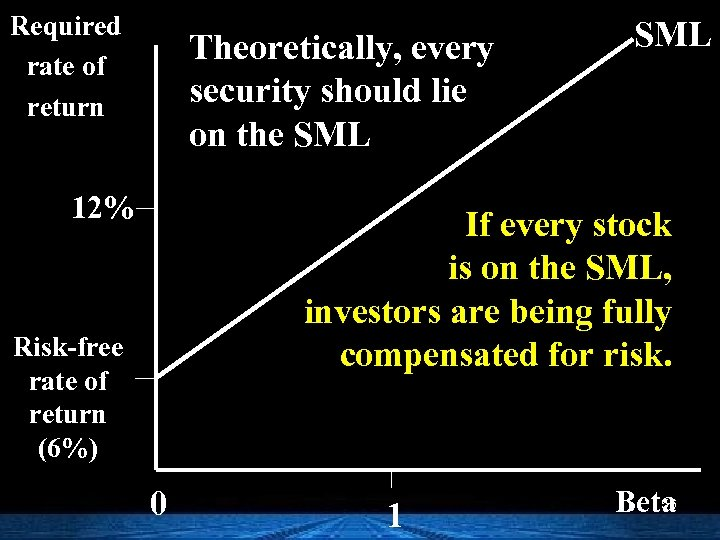Required rate of return Theoretically, every security should lie on the SML 12% SML If every stock is on the SML, investors are being fully compensated for risk. Risk-free rate of return (6%) 0 1 56 Beta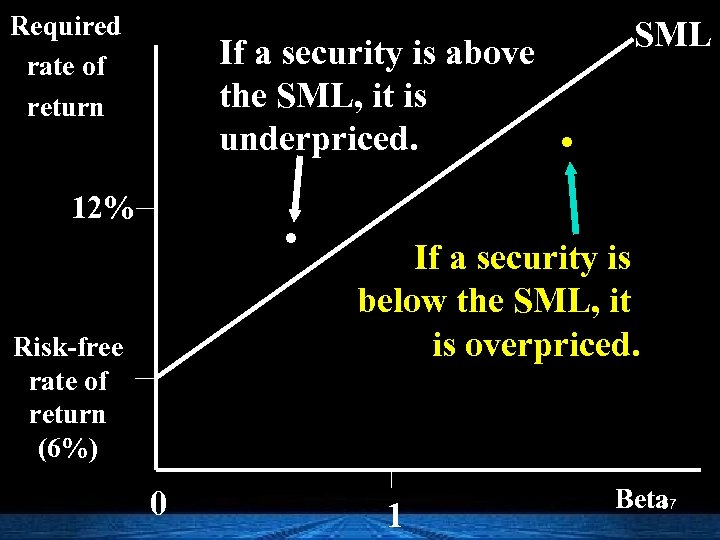Required rate of return If a security is above the SML, it is underpriced. . 12% Risk-free rate of return (6%) 0 SML . If a security is below the SML, it is overpriced. 1 Beta 57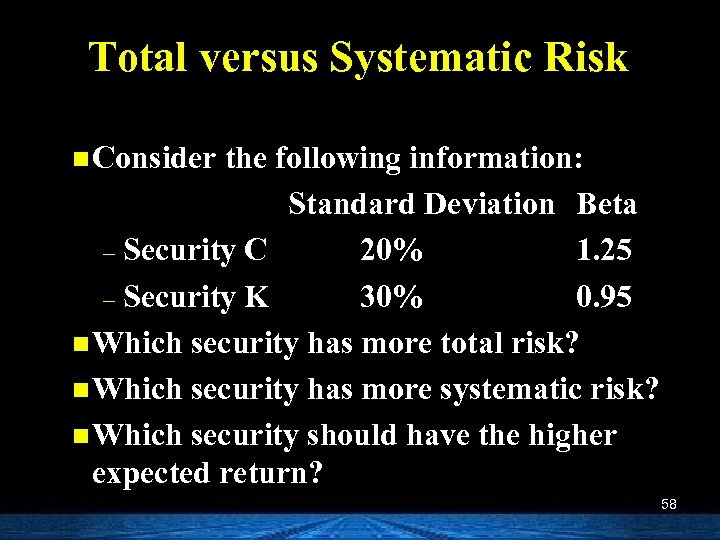Total versus Systematic Risk n Consider the following information: Standard Deviation Beta – Security C 20% 1. 25 – Security K 30% 0. 95 n Which security has more total risk? n Which security has more systematic risk? n Which security should have the higher expected return? 58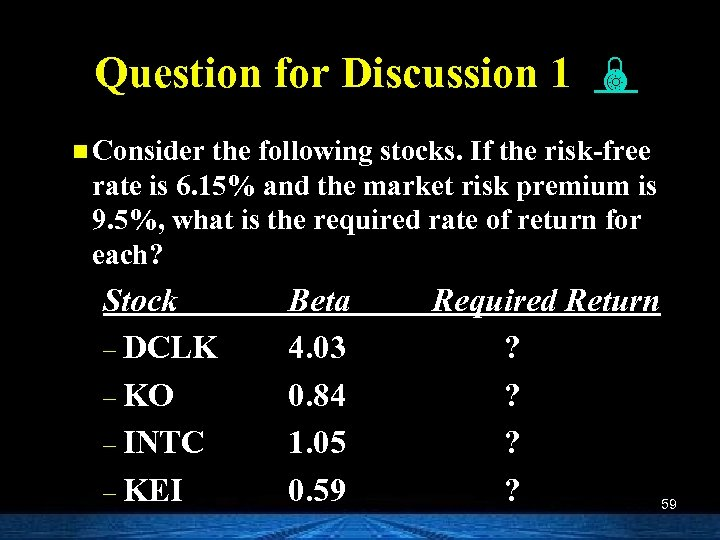Question for Discussion 1 n Consider the following stocks. If the risk-free rate is 6. 15% and the market risk premium is 9. 5%, what is the required rate of return for each? Stock – DCLK – KO – INTC – KEI Beta 4. 03 0. 84 1. 05 0. 59 Required Return ? ? 59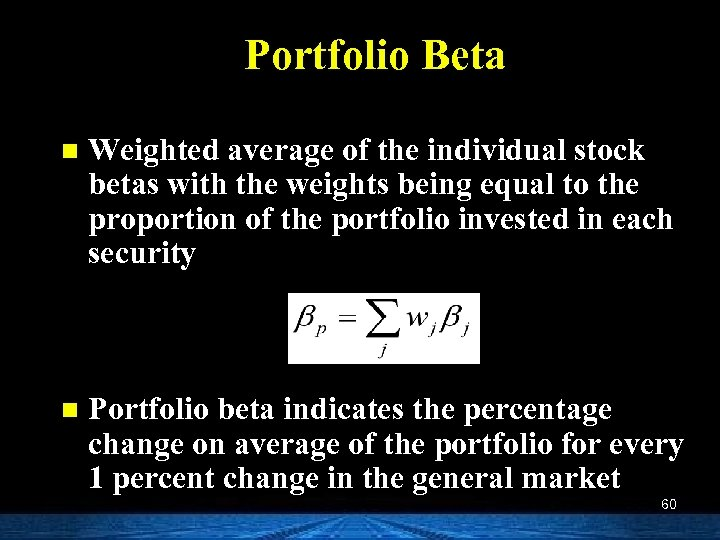Portfolio Beta n Weighted average of the individual stock betas with the weights being equal to the proportion of the portfolio invested in each security n Portfolio beta indicates the percentage change on average of the portfolio for every 1 percent change in the general market 60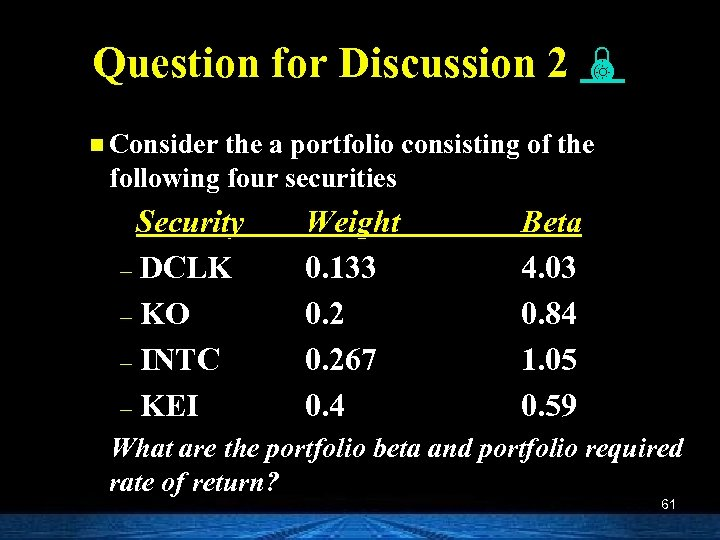Question for Discussion 2 n Consider the a portfolio consisting of the following four securities Security – DCLK – KO – INTC – KEI Weight 0. 133 0. 267 0. 4 Beta 4. 03 0. 84 1. 05 0. 59 What are the portfolio beta and portfolio required rate of return? 61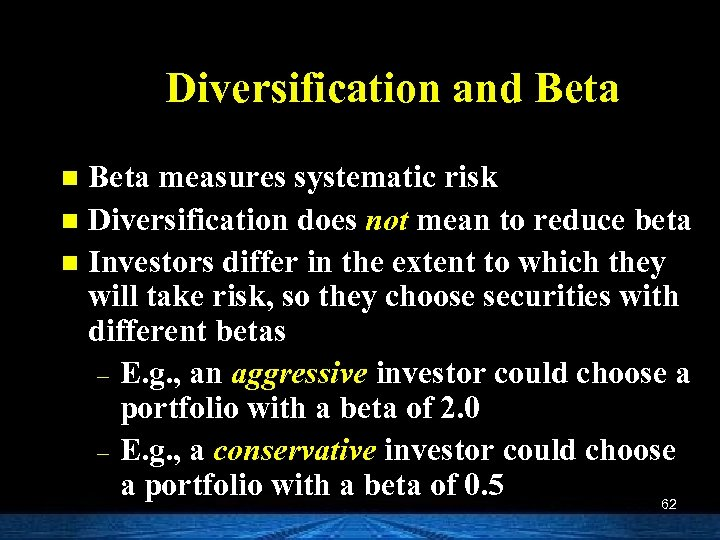Diversification and Beta measures systematic risk n Diversification does not mean to reduce beta n Investors differ in the extent to which they will take risk, so they choose securities with different betas – E. g. , an aggressive investor could choose a portfolio with a beta of 2. 0 – E. g. , a conservative investor could choose a portfolio with a beta of 0. 5 62 n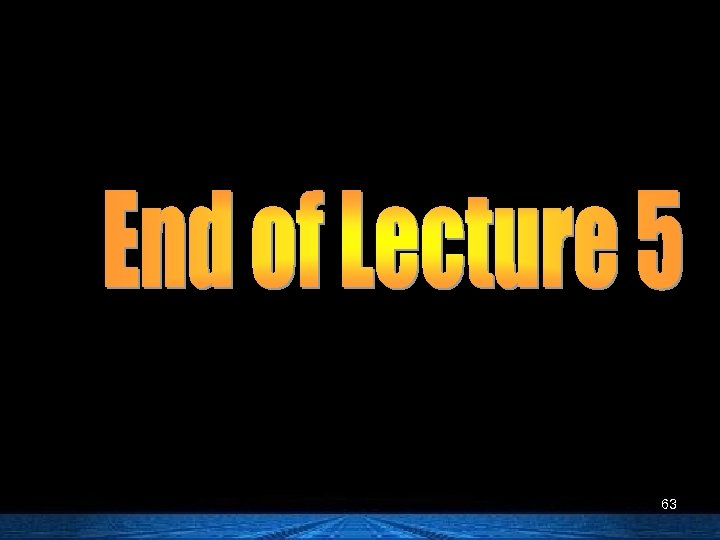63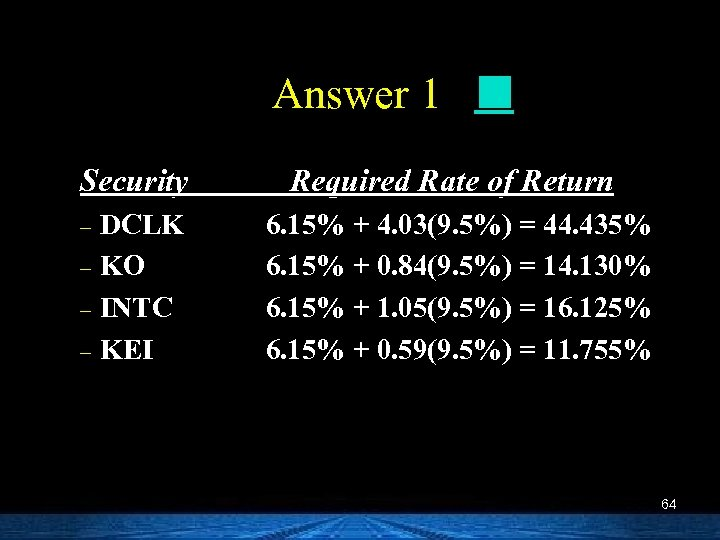Answer 1 Security Required Rate of Return DCLK – KO – INTC – KEI 6. 15% + 4. 03(9. 5%) = 44. 435% 6. 15% + 0. 84(9. 5%) = 14. 130% 6. 15% + 1. 05(9. 5%) = 16. 125% 6. 15% + 0. 59(9. 5%) = 11. 755% – 64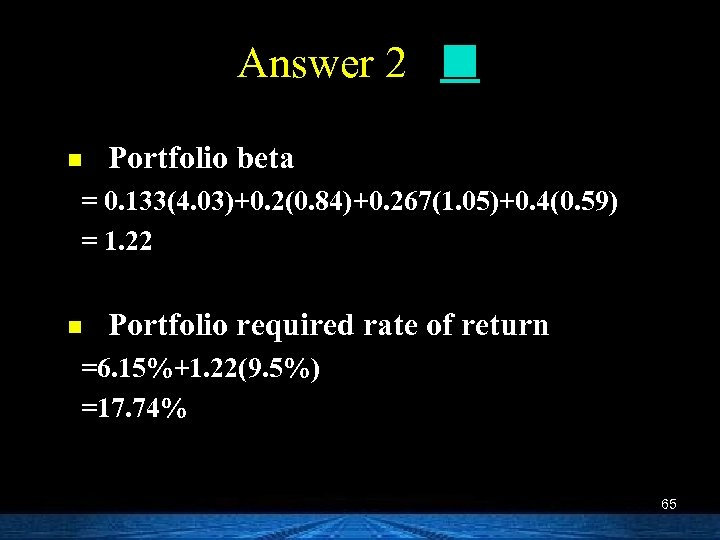Answer 2 n Portfolio beta = 0. 133(4. 03)+0. 2(0. 84)+0. 267(1. 05)+0. 4(0. 59) = 1. 22 n Portfolio required rate of return =6. 15%+1. 22(9. 5%) =17. 74% 65